Courses

# Test: Communication Systems - 8

## 25 Questions MCQ Test Communication Theory | Test: Communication Systems - 8

Description
This mock test of Test: Communication Systems - 8 for Electronics and Communication Engineering (ECE) helps you for every Electronics and Communication Engineering (ECE) entrance exam. This contains 25 Multiple Choice Questions for Electronics and Communication Engineering (ECE) Test: Communication Systems - 8 (mcq) to study with solutions a complete question bank. The solved questions answers in this Test: Communication Systems - 8 quiz give you a good mix of easy questions and tough questions. Electronics and Communication Engineering (ECE) students definitely take this Test: Communication Systems - 8 exercise for a better result in the exam. You can find other Test: Communication Systems - 8 extra questions, long questions & short questions for Electronics and Communication Engineering (ECE) on EduRev as well by searching above.
QUESTION: 1

### In a satellite system circular polarization is to be obtained. The antenna used is

Solution:

Helical antenna produces a circularly polarized radiation if its two components are at right angles and are equal. It is very commonly used in radio telemetry.

QUESTION: 2

### A horizontal output stage is cutoff for retrace and 40% of trace. If time for each horizontal line is 64 μs and retrace time is 12 μs, the transistor is conducting for about

Solution:

Total time for each horizontal line =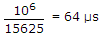.

Time for trace = 64 - 12 = 52 μs.

Time for which transistor is not conducting = 12 + 0.4 x 52 = 32.8 ms.

Time for which transistor is conducting = 64 - 32.8 = 31 μs.

QUESTION: 3

### A TV remote control works on the principle of

Solution:

Infrared light is used in TV remote control.

QUESTION: 4

As the magnitude of reverse bias is increased, the capacitance of varactor diode

Solution:

When magnitude of reverse bias is increased, thickness of depletion layer increases.

Capacitance is inversely proportional to thickness.

QUESTION: 5

Solution:

QUESTION: 6

Push pull amplifier is

Solution:

It is a class B amplifier.

QUESTION: 7

The tape recorders used in homes have

Solution:

Common head and common amplifier is used.

QUESTION: 8

In a communication system, noise is most likely to affect the signal

Solution:

Noise is mostly generated in channel.

QUESTION: 9

Each frequency in the modulating wave gives rise to four side bands.

Solution:

Each frequency gives two side bands.

QUESTION: 10

Assertion (A): CCIR-B system has 625 scanning lines per frame and 2 : 1 interlace.

Reason (R): The aspect ratio is 4 : 3

Solution: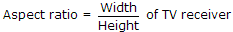.

QUESTION: 11

In VHS format each video head is tilted from azimuth by

Solution:

Tilting of heads is done to reduce cross-talk.

QUESTION: 12

The number of possible colours with a 4 line digital input monitor are

Solution:

24 = 16.

QUESTION: 13

Which of the following is a better recording medium?

Solution:

Chromium di oxide has very good retentivity.

QUESTION: 14

Convolution of x(t + 5) with impulse function δ(t - 7) is equal to

Solution:

Convolution of | x(t + s) with d(t - 7)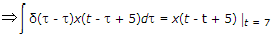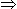x(t - 7 + 5)x(t - 2).

QUESTION: 15

An angle modulated signal is given by s(t) = cos 2p (2 x 106t + 30 sin 150t + 40 cos 150t). The maximum frequency and phase deviations of s(t) are

Solution:

s(t) = cos 2p(2 x 10+16t + 30 sin 150t + 40 cos 150t)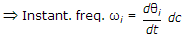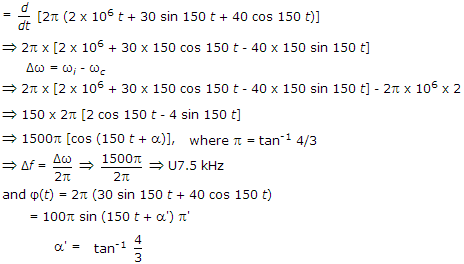Phase deviation = 100 p

Frequency deviation = 7.5 kHz.

QUESTION: 16

The PSD and the power of a signal g(t) are respectively Sg(ω) and Pg, the PSD and the power of signal a.g(t) are respectively

Solution:
QUESTION: 17

The simplest method of suppression of unwanted side band in AM is

Solution:

Unwanted frequency is removed by filtering.

QUESTION: 18

In a 100% amplitude modulated signal, the power in the upper sideband when carrier power is to be 100 W and modulation system SSBSC, is

Solution:

Modulation index = 100%1

Pc = 100 W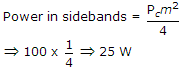.

QUESTION: 19

For attenuation of low frequencies we should use

Solution:

Series capacitance has high reactance for low frequencies.

QUESTION: 20

Assertion (A): A colour TV uses a SAW filter to give stable band pass response and high gain.

Reason (R): Devices using SAW principle are used in many systems.

Solution:

SAW means Surface Acoustic Wave. SAW filter is very commonly used in TV sets.

QUESTION: 21

At night the ionosphere can be considered to be consisting of

Solution:

During night D layer is almost absent. Moreover F1 and F2 layers merge to form single F2 layer. Hence only D and F2 layers at night.

QUESTION: 22

Which one of the following is true?

Solution:

Z0 for twin wire cable is about 300 ohms while that for coaxial cable is about 75 ohms.

QUESTION: 23

A balun transformer gives an impedance transformation of

Solution:

Balun gives 4 : 1 impedance transformation and can be used to interconnect twin wire feeder cable to coaxial cable.

QUESTION: 24

The EHT voltage in a picture tube of TV is

Solution:

EHT voltage is 1 kV for each diagonal inch of CRT screen.

QUESTION: 25

A 1000 kHz carrier wave modulated 40% at 40000 Hz is applied to a resonant circuit tuned to a carrier frequency and having Q = 140. What is the degree of modulator after transmission through the circuit?

Solution:

m0 = m(1 + 4Q2δ2)1/2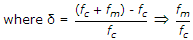.